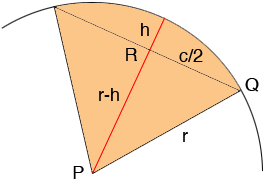SEARCH HOMEMath Central Quandaries & QueriesSubject: finding a radius Name: Kevin Who are you: Other I have the length of the cord and the distance from the cord to the arc, is it possible to find the radius with just these parameters?Kevin,

I let c be the length of the cord, h be the height of the arc above the centre of the cord and r be the radius of the circle.In the diagram the triangle PQR is a right triangle and hence by Pythagoras' Theorem

(c/2)2 + (r - h)2 = r2

Simplifying this becomes

c2/4 + r2 - 2hr + h2 = r2 or
r = (4h2 + c2)/(8h)

PennyMath Central is supported by the University of Regina and The Pacific Institute for the Mathematical Sciences.